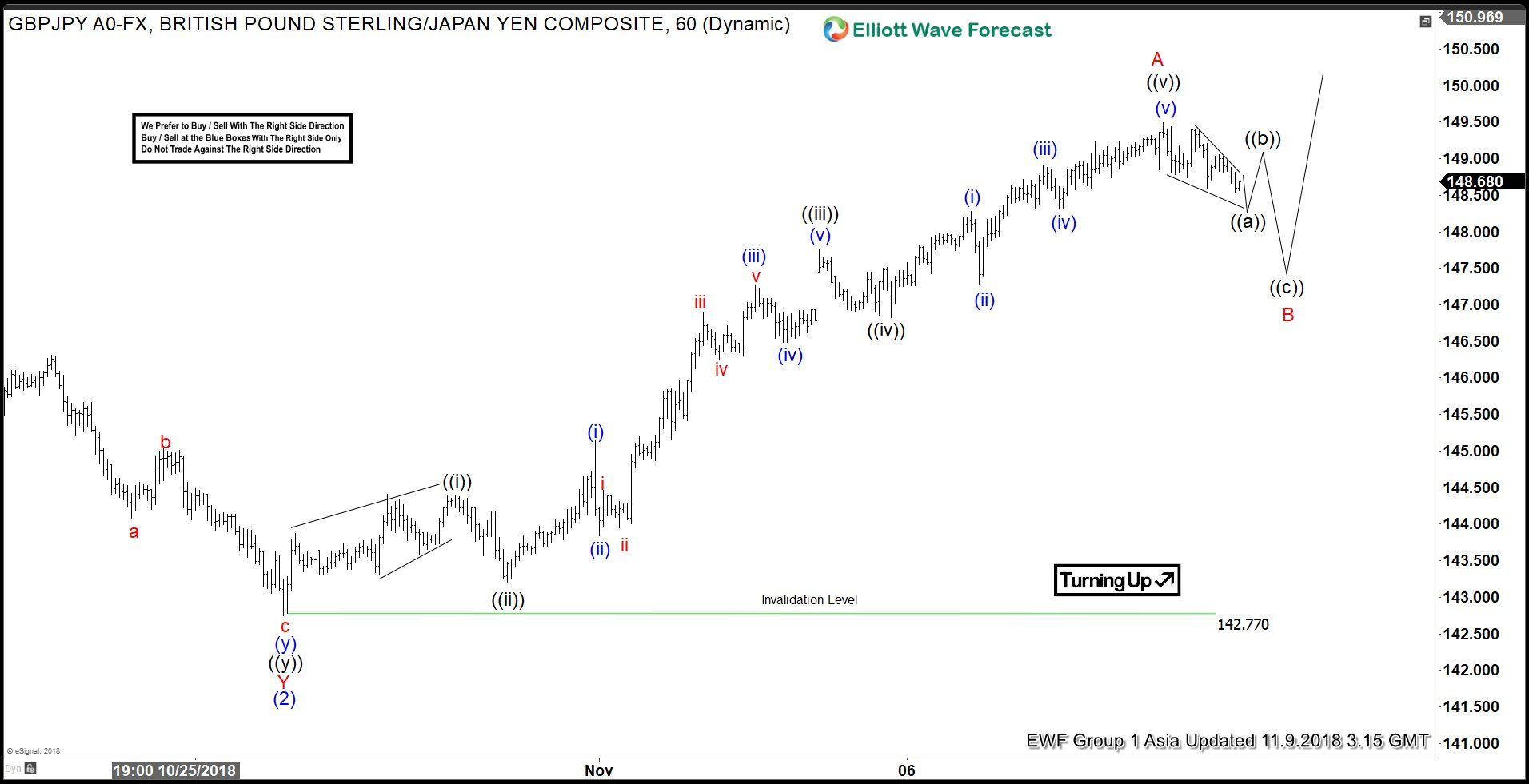# GBPJPY in Correction Before Next Leg Higher

Short Term Elliott wave view in GBPJPY suggests that the decline to 142.77 ended wave (2) in Intermediate degree. Up from there, the pair rallies as an impulse and ended Minor wave A at 149.49. Minor wave B pullback is currently in progress to correct cycle from Oct 26 low (142.77) before the next leg higher. We can see internals of wave A unfolded in 5 waves where Minute wave ((i)) ended at 144.39, Minute wave ((ii)) ended at 143.19, Minute wave ((iii)) ended at 147.77, Minute wave ((iv)) ended at 146.83, and Minute wave ((v)) ended at 149.49

Subdivision of Minute wave ((iii)) and ((v)) further reveals another 5 waves of a lesser Minutte degree (blue color), fulfilling the rule of an impulse wave. This rally from 142.77 low to 149.49 high completed Minor wave A of a larger degree and ended cycle from Oct 26 low. Pair should now pullback in Minor wave B to correct this cycle in the sequence of 3, 7, or 11 swing before the rally resumes, provided that pivot at 142.77 low stays intact.

Minor wave B pullback is currently proposed to take the form of a zigzag where Minute wave ((a)) of B is in progress as a diagonal. Afterwards, pair should bounce in Minute wave ((b)) of B before turning lower in Minute wave ((c)) of B. We can measure potential target for Minor wave B pullback with more precision once Minute wave ((a)) and ((b)) swing have formed. The potential target for Minute wave ((c)) of B should be at the area of 100% – 123.6% fibonacci extension of Minute wave ((a)) of B.

A rough estimate of 50 – 61.8% fibonacci retracement can be used for the time being  to estimate the Minor wave B target while waiting for the Minute wave ((a)) and ((b)) to fully form. A 50 – 61.8% Fibonacci retracement of Minor wave A comes at 145.3 – 146.1 for potential support area where Minor wave B pullback can complete. We don’t like selling the pullback and expect buyers to appear again when the Minor wave B pullback is complete in 3-7-11 swing.

#### GBPJPY 1 Hour Elliott Wave Chart